Search Results
• #### Determine the inverse Laplace transform of the function: F(s) = [10(s + 3)(s + 5)]/[s(s +...

Determine the inverse Laplace transform of the function: F(s) = [10(s + 3)(s + 5)]/[s(s + 1)(s + 6)] and build a plot as a function of time. Step by step neatly please. thanks

• #### 8.5 Consider a system with transfer function ĝ(s) = (s – 1)(s+2) (s + 1)(s –...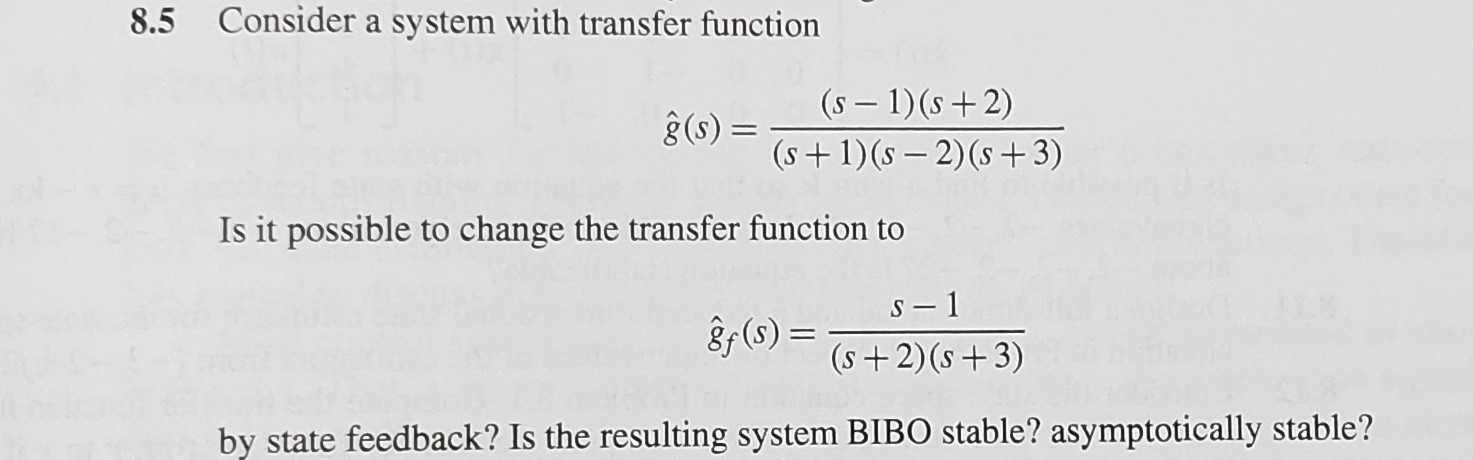8.5 Consider a system with transfer function ĝ(s) = (s – 1)(s+2) (s + 1)(s – 2)(s +3) Is it possible to change the transfer function to S-1 8f(s) =_ (s + 2)(s +3) by state feedback? Is the resulting system BIBO stable? asymptotically stable?

• #### Consider the following data. Mg(s) + S(s) MgS(s) H = -598.0 kJ SO2(g) S(s) + O2(g)...

Consider the following data. Mg(s) + S(s) MgS(s) H = -598.0 kJ SO2(g) S(s) + O2(g) H = +296.8 kJ MgS(s) + 2 MgO(s) 3 Mg(s) + SO2(g) H = +1504.6 kJ Use Hess's law to calculate H for the reaction below. 2 Mg(s) + O2(g) 2 MgO(s)

• #### E(s) In the figure below, find the transfer function G(s) = N(s) N(s) Y(s) 10 E(s)...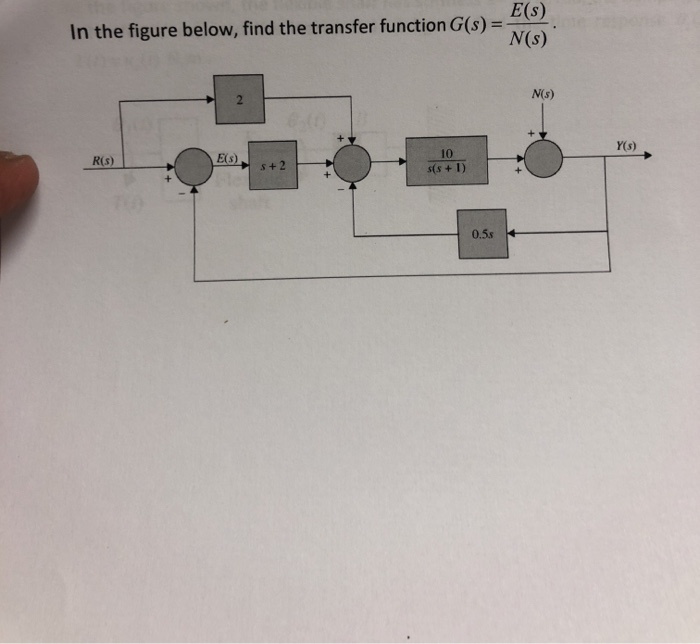E(s) In the figure below, find the transfer function G(s) = N(s) N(s) Y(s) 10 E(s) R(s) s+2 s(s + 1) 0.5s E(s) In the figure below, find the transfer function G(s) = N(s) N(s) Y(s) 10 E(s) R(s) s+2 s(s + 1) 0.5s

• #### 0.1 G(S)(s+10)(s + 0.1) Bode plot 0.1 G(S)(s+10)(s + 0.1) Bode plot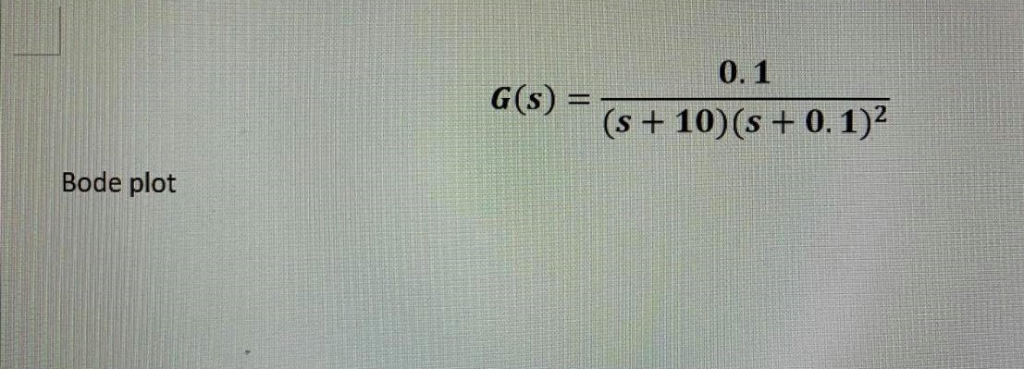0.1 G(S)(s+10)(s + 0.1) Bode plot 0.1 G(S)(s+10)(s + 0.1) Bode plot

• #### Consi der the control system illustrated in Figure P. 2 C(s) R(s) s t Ge(s) s(s...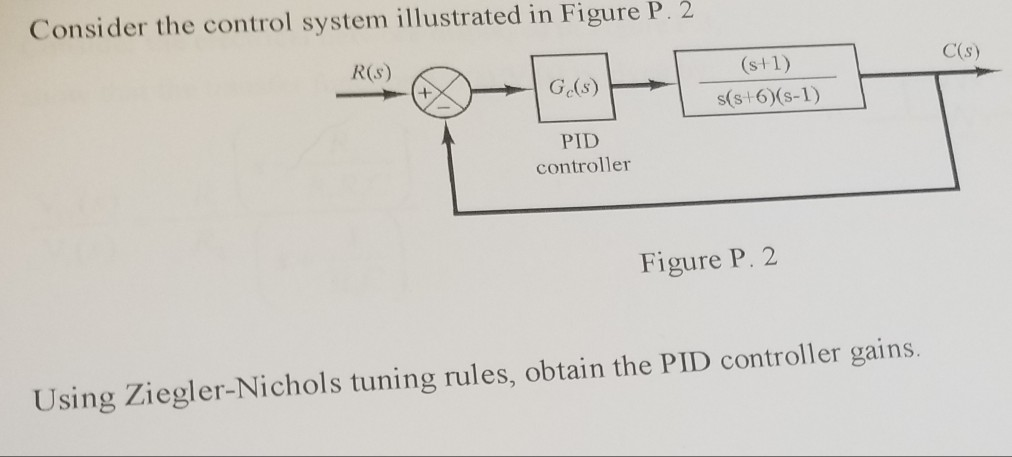Consi der the control system illustrated in Figure P. 2 C(s) R(s) s t Ge(s) s(s 6)(s-1) PID controller Figure P. 2 Using Ziegler-Nichols tuning rules, obtain the PID controller gains.

• #### Go(s) R(S) + Gr(s) Ge(s) Gy(s) H(s) Reduce the block diagram shown to a single transfer...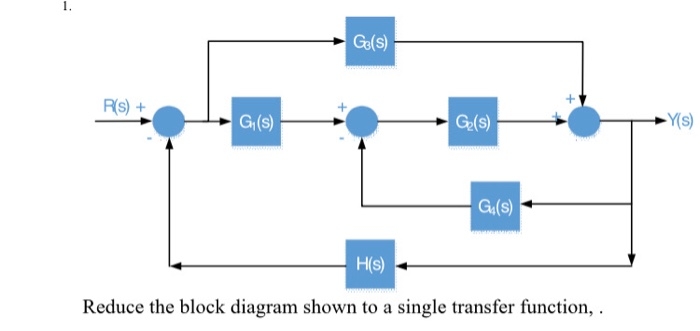Go(s) R(S) + Gr(s) Ge(s) Gy(s) H(s) Reduce the block diagram shown to a single transfer function,

• #### 2nd. In the control system below; w(s) + R(s) E(s) Y(s) Ko+Kp.s s(s+1) Sistem a. The...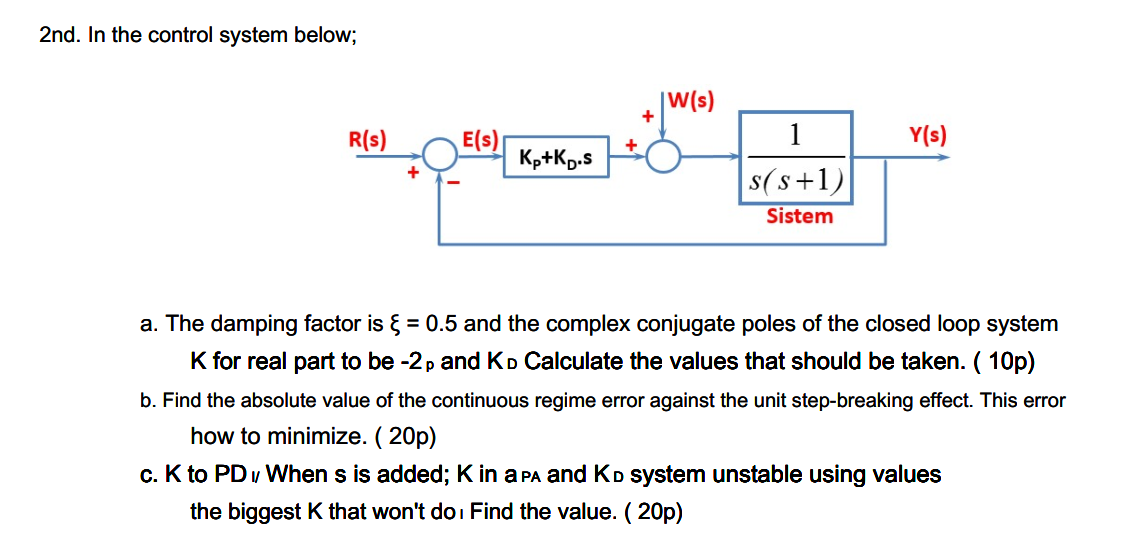2nd. In the control system below; w(s) + R(s) E(s) Y(s) Ko+Kp.s s(s+1) Sistem a. The damping factor is & = 0.5 and the complex conjugate poles of the closed loop system K for real part to be -2p and Ko Calculate the values that should be taken. ( 10p) b. Find the absolute value of the continuous regime error...

• #### Problems: Consider the following system, G11(s) G12(8) G21 (s) G22(s) S+I S+2 G(s) 1S+2 s+1 and...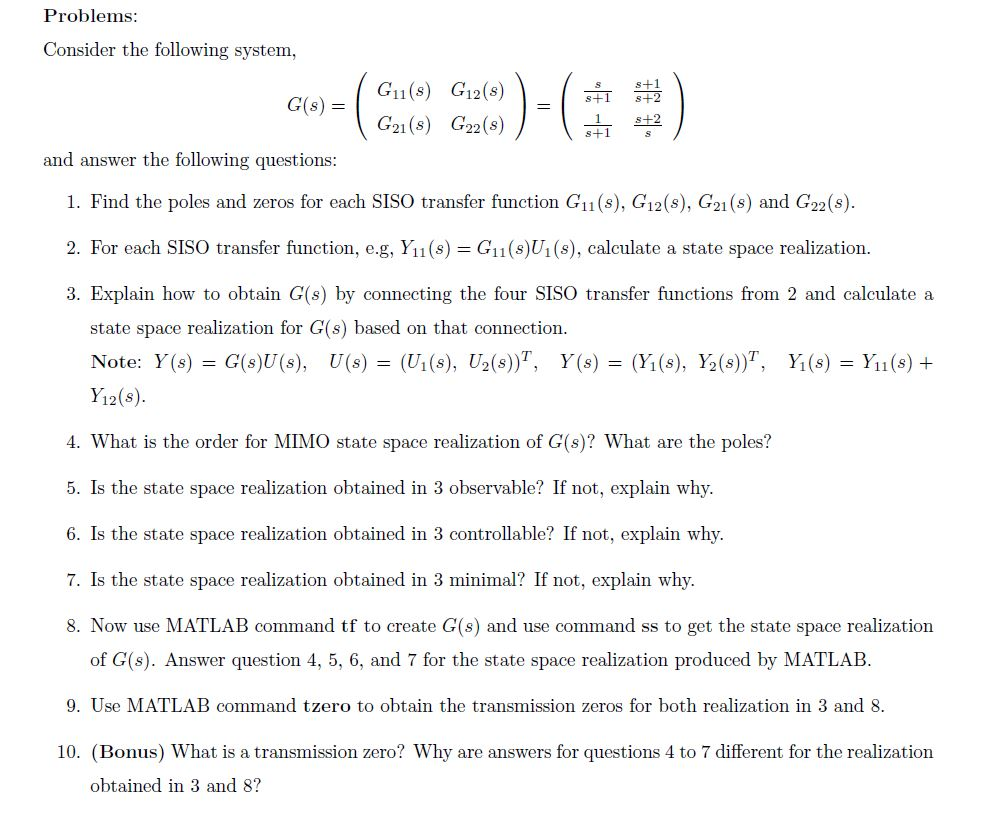Problems: Consider the following system, G11(s) G12(8) G21 (s) G22(s) S+I S+2 G(s) 1S+2 s+1 and answer the following questions 1. Find the poles and zeros for each SISO transfer function G1 (s), G12(s), G21 (s) and G22(s) 2. For each SISO transfer function, eg, Yu (s) = G11(s)U1 (s), calculate a state space realization. 3. Explain how to obtain...

• #### reagent(s); OH reagent(s); -OH reagent(s); COH reagent(s);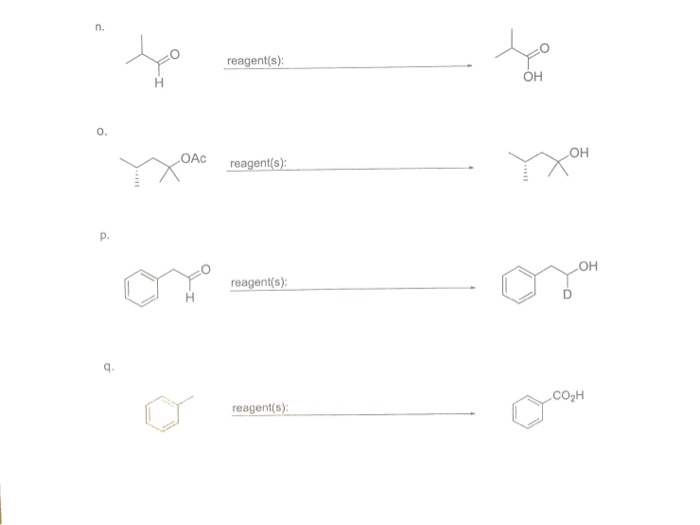reagent(s); OH reagent(s); -OH reagent(s); COH reagent(s);